# Drawing Pattern in C++

## Drawing Pattern in C++ using Nested Loops:

In this article, I am going to discuss how to draw Patterns using Nested Loops in C++ Language with examples. Please read our previous articles, where we discussed Nested Loops in C++ with examples. Here, we will learn how to draw patterns, different patterns we can draw easily using nested ‘for’ loop.

##### Drawing Pattern in C++

We will display the following pattern: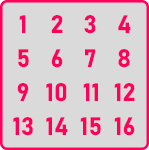It is nothing but a 2D array. So, we can write it as,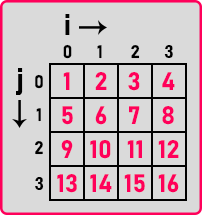Now let us look at the program of this pattern.

##### Program to Print Pattern 1:
```#include <iostream>
using namespace std;
int main()
{
int n, count = 0;
cout << "Enter Number: ";
cin >> n;
for (int i = 0; i < n; i++)
{
for (int j = 0; j < n; j++)
{
count++;
cout << count << " ";
}
cout << endl;
}
}
```
###### Output: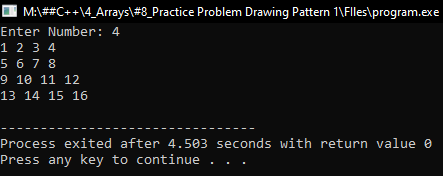##### Drawing Pattern 2:

Now, we will display the following pattern: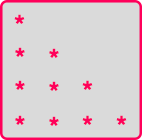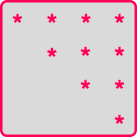Let us write the program for,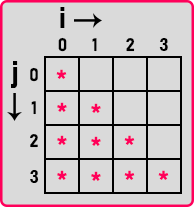##### Program to Print Pattern 2a:
```#include <iostream>
using namespace std;
int main()
{
int n;
cout << "Enter Number: ";
cin >> n;
for (int i = 0; i < n; i++)
{
for (int j = 0; j <= i; j++)
{
cout << "* ";
}
cout << endl;
}
}
```
###### Output: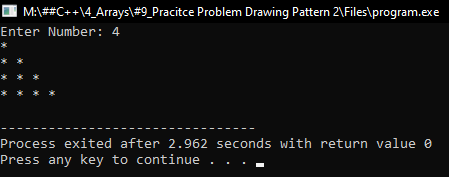Now let us write the program for,##### Program to Print Pattern 2b:
```#include <iostream>
using namespace std;
int main()
{
int n;
cout << "Enter Number: ";
cin >> n;
for (int i = 0; i < n; i++)
{
for (int j = 0; j < n; j++)
{
if (i > j)
{
cout << "  ";
}
else
{
cout << "* ";
}
}
cout << endl;
}
}
```
###### Output: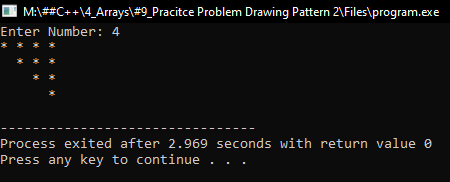##### Drawing Pattern 3:

Now we will display the following pattern: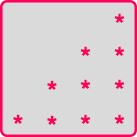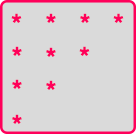Let us write the program for,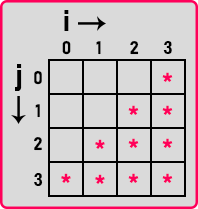##### Program to Print Pattern 3a:
```#include <iostream>
using namespace std;
int main()
{
int n, count = 0;
cout <<"Enter Number: ";
cin >> n;
for(int i = 0; i < n; i++)
{
for(int j = 0; j < n; j++)
{
if(i + j >= n - 1)
cout << "* ";
else
cout << "  ";
}
cout << endl;
}
}
```
###### Output: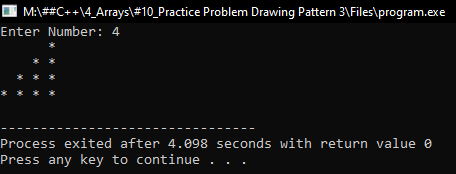Now let us write the program for,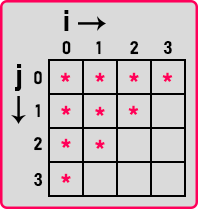##### Program to Print Pattern 3b:
```#include <iostream>
using namespace std;
int main()
{
int n, count = 0;
cout << "Enter Number: ";
cin >> n;
for (int i = 0; i < n; i++)
{
for (int j = 0; j < n; j++)
{
if (i + j <= n - 1)
cout << "* ";
else
cout << "  ";
}
cout << endl;
}
}
```
###### Output: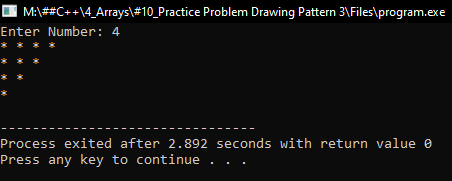1.